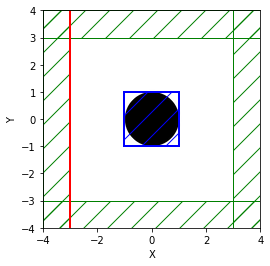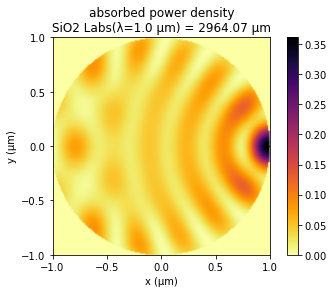# Absorbed Power Density Map of a Lossy Cylinder¶

The dft_flux routines (add_flux) described in the previous examples compute the total power in a given region (FluxRegion). It is also possible to compute the local (i.e., position-dependent) absorbed power density in a dispersive (lossy) material. This quantity is useful for obtaining a spatial map of the photon absorption. The absorbed power density is defined as $$\mathrm{Re}\, \left[ {\mathbf{E}^* \cdot \frac{d\mathbf{P}}{dt}} \right]$$ where $\mathbf{P}$ is the total polarization field. In the Fourier (frequency) domain with time-harmonic fields, this expression is $$\mathrm{Re}\, \left[ {\mathbf{E}^* \cdot (-i \omega \mathbf{P})} \right] = \omega\, \mathrm{Im}\, \left[ {\mathbf{E}^* \cdot \mathbf{P}} \right]$$ where $\mathbf{E}^* \cdot \mathbf{P}$ denotes the dot product of the complex conjugate of $\mathbf{E}$ with $\mathbf{P}$. However, since $\mathbf{D}=\mathbf{E}+\mathbf{P}$, this is equivalent to $$\omega\, \mathrm{Im}\, \left[ {\mathbf{E}^* \cdot (\mathbf{D}-\mathbf{E})} \right] = \omega\, \mathrm{Im}\, \left[ {\mathbf{E}^* \cdot \mathbf{D}} \right]$$ since $\mathbf{E}^* \cdot \mathbf{E} = |\mathbf{E}|^2$ is purely real. Calculating this quantity involves two steps: (1) compute the Fourier-transformed $\mathbf{E}$ and $\mathbf{D}$ fields in a region via the dft_fields feature and (2) in post processing, compute $\omega\, \mathrm{Im}\, \left[ {\mathbf{E}^* \cdot \mathbf{D}} \right]$.

This tutorial example involves computing the absorbed power density for a two-dimensional cylinder (radius: 1 μm) of silicon dioxide (SiO2, from the materials library) at a wavelength of 1 μm given an incident $E_z$-polarized planewave. (The attenuation length of SiO2 at this wavelength is $\lambda/\mathrm{Im}\, \sqrt{\varepsilon}$ = ~3000 μm.) We will also verify that the total power absorbed by the cylinder obtained by integrating the absorbed power density over the entire cylinder is equivalent to the same quantity computed using the alternative method involving a closed, four-sided dft_flux box (Poynting's theorem).

In :
import numpy as np
import matplotlib.pyplot as plt

import meep as mp
from meep.materials import SiO2

resolution = 100  # pixels/um

dpml = 1.0
pml_layers = [mp.PML(thickness=dpml)]

r = 1.0     # radius of cylinder
dair = 2.0  # air padding thickness

s = 2*(dpml+dair+r)
cell_size = mp.Vector3(s,s)

wvl = 1.0
fcen = 1/wvl

# is_integrated=True necessary for any planewave source extending into PML
sources = [mp.Source(mp.GaussianSource(fcen,fwidth=0.1*fcen,is_integrated=True),
center=mp.Vector3(-0.5*s+dpml),
size=mp.Vector3(0,s),
component=mp.Ez)]

symmetries = [mp.Mirror(mp.Y)]

geometry = [mp.Cylinder(material=SiO2,
center=mp.Vector3(),
height=mp.inf)]

sim = mp.Simulation(resolution=resolution,
cell_size=cell_size,
boundary_layers=pml_layers,
sources=sources,
k_point=mp.Vector3(),
symmetries=symmetries,
geometry=geometry)

fcen,0,1,
center=mp.Vector3(),
size=mp.Vector3(2*r,2*r),
yee_grid=True)

# closed box surrounding cylinder for computing total incoming flux
mp.FluxRegion(center=mp.Vector3(x=-r),size=mp.Vector3(0,2*r),weight=+1),
mp.FluxRegion(center=mp.Vector3(x=+r),size=mp.Vector3(0,2*r),weight=-1),
mp.FluxRegion(center=mp.Vector3(y=+r),size=mp.Vector3(2*r,0),weight=-1),
mp.FluxRegion(center=mp.Vector3(y=-r),size=mp.Vector3(2*r,0),weight=+1))

sim.run(until_after_sources=100)

Dz = sim.get_dft_array(dft_fields,mp.Dz,0)
Ez = sim.get_dft_array(dft_fields,mp.Ez,0)
absorbed_power_density = 2*np.pi*fcen * np.imag(np.conj(Ez)*Dz)

dxy = 1/resolution**2
absorbed_power = np.sum(absorbed_power_density)*dxy
absorbed_flux = mp.get_fluxes(flux_box)
err = abs(absorbed_power-absorbed_flux)/absorbed_flux
print("flux:, {} (dft_fields), {} (dft_flux), {} (error)".format(absorbed_power,absorbed_flux,err))

-----------
Initializing structure...
Halving computational cell along direction y
time for choose_chunkdivision = 0.00278592 s
Working in 2D dimensions.
Computational cell is 8 x 8 x 0 with resolution 100
cylinder, center = (0,0,0)
radius 1, height 1e+20, axis (0, 0, 1)
dielectric constant epsilon diagonal = (1,1,1)
time for set_epsilon = 0.94641 s
lorentzian susceptibility: frequency=9.67865, gamma=0.0806554
-----------

Meep progress: 3.24/200.0 = 1.6% done in 4.0s, 243.3s to go
on time step 650 (time=3.25), 0.00616112 s/step
Meep progress: 6.595/200.0 = 3.3% done in 8.0s, 234.8s to go
on time step 1322 (time=6.61), 0.00595972 s/step
Meep progress: 10.0/200.0 = 5.0% done in 12.0s, 228.2s to go
on time step 2003 (time=10.015), 0.00587584 s/step
Meep progress: 13.16/200.0 = 6.6% done in 16.0s, 227.3s to go
on time step 2635 (time=13.175), 0.00632952 s/step
Meep progress: 16.52/200.0 = 8.3% done in 20.0s, 222.3s to go
on time step 3308 (time=16.54), 0.00595308 s/step
Meep progress: 19.39/200.0 = 9.7% done in 24.0s, 223.7s to go
on time step 3882 (time=19.41), 0.00697273 s/step
Meep progress: 22.77/200.0 = 11.4% done in 28.0s, 218.1s to go
on time step 4559 (time=22.795), 0.00591308 s/step
Meep progress: 26.085/200.0 = 13.0% done in 32.0s, 213.5s to go
on time step 5223 (time=26.115), 0.00603051 s/step
Meep progress: 29.465/200.0 = 14.7% done in 36.0s, 208.5s to go
on time step 5898 (time=29.49), 0.00592621 s/step
Meep progress: 32.87/200.0 = 16.4% done in 40.0s, 203.5s to go
on time step 6579 (time=32.895), 0.00587512 s/step
Meep progress: 36.265/200.0 = 18.1% done in 44.0s, 198.8s to go
on time step 7258 (time=36.29), 0.00589753 s/step
Meep progress: 39.63/200.0 = 19.8% done in 48.0s, 194.4s to go
on time step 7931 (time=39.655), 0.00595055 s/step
Meep progress: 43.075/200.0 = 21.5% done in 52.0s, 189.6s to go
on time step 8621 (time=43.105), 0.00580339 s/step
Meep progress: 46.385/200.0 = 23.2% done in 56.0s, 185.6s to go
on time step 9282 (time=46.41), 0.00605481 s/step
Meep progress: 49.795/200.0 = 24.9% done in 60.0s, 181.1s to go
on time step 9965 (time=49.825), 0.00586147 s/step
Meep progress: 53.120000000000005/200.0 = 26.6% done in 64.0s, 177.1s to go
on time step 10629 (time=53.145), 0.0060261 s/step
Meep progress: 56.52/200.0 = 28.3% done in 68.1s, 172.8s to go
on time step 11309 (time=56.545), 0.00588784 s/step
Meep progress: 59.885/200.0 = 29.9% done in 72.1s, 168.6s to go
on time step 11982 (time=59.91), 0.00594881 s/step
Meep progress: 63.22/200.0 = 31.6% done in 76.1s, 164.6s to go
on time step 12649 (time=63.245), 0.0059984 s/step
Meep progress: 66.57000000000001/200.0 = 33.3% done in 80.1s, 160.5s to go
on time step 13319 (time=66.595), 0.0059716 s/step
Meep progress: 69.935/200.0 = 35.0% done in 84.1s, 156.3s to go
on time step 13993 (time=69.965), 0.00593998 s/step
Meep progress: 73.265/200.0 = 36.6% done in 88.1s, 152.3s to go
on time step 14659 (time=73.295), 0.00600958 s/step
Meep progress: 76.64/200.0 = 38.3% done in 92.1s, 148.2s to go
on time step 15335 (time=76.675), 0.00592433 s/step
Meep progress: 80.035/200.0 = 40.0% done in 96.1s, 144.0s to go
on time step 16013 (time=80.065), 0.00590122 s/step
Meep progress: 83.44/200.0 = 41.7% done in 100.1s, 139.8s to go
on time step 16694 (time=83.47), 0.00587495 s/step
Meep progress: 86.82000000000001/200.0 = 43.4% done in 104.1s, 135.7s to go
on time step 17371 (time=86.855), 0.00591555 s/step
Meep progress: 90.22500000000001/200.0 = 45.1% done in 108.1s, 131.5s to go
on time step 18052 (time=90.26), 0.00587912 s/step
Meep progress: 93.58500000000001/200.0 = 46.8% done in 112.1s, 127.5s to go
on time step 18723 (time=93.615), 0.00596502 s/step
Meep progress: 96.95/200.0 = 48.5% done in 116.1s, 123.4s to go
on time step 19397 (time=96.985), 0.00593889 s/step
Meep progress: 100.32000000000001/200.0 = 50.2% done in 120.1s, 119.3s to go
on time step 20071 (time=100.355), 0.00594026 s/step
Meep progress: 103.68/200.0 = 51.8% done in 124.1s, 115.3s to go
on time step 20743 (time=103.715), 0.00596124 s/step
Meep progress: 107.11500000000001/200.0 = 53.6% done in 128.1s, 111.1s to go
on time step 21430 (time=107.15), 0.00582342 s/step
Meep progress: 110.525/200.0 = 55.3% done in 132.1s, 106.9s to go
on time step 22113 (time=110.565), 0.00586115 s/step
Meep progress: 113.855/200.0 = 56.9% done in 136.1s, 103.0s to go
on time step 22779 (time=113.895), 0.00601218 s/step
Meep progress: 117.17/200.0 = 58.6% done in 140.1s, 99.0s to go
on time step 23442 (time=117.21), 0.00604035 s/step
Meep progress: 120.55/200.0 = 60.3% done in 144.1s, 95.0s to go
on time step 24119 (time=120.595), 0.00591443 s/step
Meep progress: 123.93/200.0 = 62.0% done in 148.1s, 90.9s to go
on time step 24795 (time=123.975), 0.00591771 s/step
Meep progress: 127.345/200.0 = 63.7% done in 152.1s, 86.8s to go
on time step 25478 (time=127.39), 0.00586339 s/step
Meep progress: 130.695/200.0 = 65.3% done in 156.1s, 82.8s to go
on time step 26148 (time=130.74), 0.00597442 s/step
Meep progress: 134.075/200.0 = 67.0% done in 160.1s, 78.7s to go
on time step 26824 (time=134.12), 0.005918 s/step
Meep progress: 137.465/200.0 = 68.7% done in 164.1s, 74.7s to go
on time step 27502 (time=137.51), 0.005903 s/step
Meep progress: 140.85/200.0 = 70.4% done in 168.1s, 70.6s to go
on time step 28179 (time=140.895), 0.00591219 s/step
Meep progress: 144.24/200.0 = 72.1% done in 172.1s, 66.5s to go
on time step 28858 (time=144.29), 0.00590082 s/step
Meep progress: 147.625/200.0 = 73.8% done in 176.1s, 62.5s to go
on time step 29535 (time=147.675), 0.0059085 s/step
Meep progress: 151.025/200.0 = 75.5% done in 180.1s, 58.4s to go
on time step 30216 (time=151.08), 0.00587643 s/step
Meep progress: 154.425/200.0 = 77.2% done in 184.1s, 54.3s to go
on time step 30896 (time=154.48), 0.00588855 s/step
Meep progress: 157.82500000000002/200.0 = 78.9% done in 188.1s, 50.3s to go
on time step 31577 (time=157.885), 0.00588064 s/step
Meep progress: 161.245/200.0 = 80.6% done in 192.1s, 46.2s to go
on time step 32261 (time=161.305), 0.00585296 s/step
Meep progress: 164.605/200.0 = 82.3% done in 196.1s, 42.2s to go
on time step 32933 (time=164.665), 0.00595906 s/step
Meep progress: 167.955/200.0 = 84.0% done in 200.1s, 38.2s to go
on time step 33603 (time=168.015), 0.00597094 s/step
Meep progress: 171.31/200.0 = 85.7% done in 204.2s, 34.2s to go
on time step 34275 (time=171.375), 0.00596032 s/step
Meep progress: 174.695/200.0 = 87.3% done in 208.2s, 30.2s to go
on time step 34953 (time=174.765), 0.00590648 s/step
Meep progress: 178.01500000000001/200.0 = 89.0% done in 212.2s, 26.2s to go
on time step 35617 (time=178.085), 0.00602658 s/step
Meep progress: 181.37/200.0 = 90.7% done in 216.2s, 22.2s to go
on time step 36288 (time=181.44), 0.00597098 s/step
Meep progress: 184.735/200.0 = 92.4% done in 220.2s, 18.2s to go
on time step 36961 (time=184.805), 0.0059467 s/step
Meep progress: 188.035/200.0 = 94.0% done in 224.2s, 14.3s to go
on time step 37621 (time=188.105), 0.00606458 s/step
Meep progress: 191.455/200.0 = 95.7% done in 228.2s, 10.2s to go
on time step 38307 (time=191.535), 0.00583756 s/step
Meep progress: 194.79/200.0 = 97.4% done in 232.2s, 6.2s to go
on time step 38973 (time=194.865), 0.00600972 s/step
Meep progress: 198.16/200.0 = 99.1% done in 236.2s, 2.2s to go
on time step 39649 (time=198.245), 0.00592657 s/step
run 0 finished at t = 200.0 (40000 timesteps)
flux:, 0.13120421825956843 (dft_fields), 0.13249534167200247 (dft_flux), 0.0097446702362583 (error)


There is one important item to note: in order to eliminate discretization artifacts when computing the $\mathbf{E}^* \cdot \mathbf{D}$ dot-product, the add_dft_fields definition includes yee_grid=True which ensures that the $E_z$ and $D_z$ fields are computed on the Yee grid rather than interpolated to the centered grid. As a corollary, we cannot use get_array_metadata to obtain the coordinates of the dft_fields region or its interpolation weights because this involves the centered grid.

The two values for the total absorbed power which are displayed at the end of the run are nearly equivalent. The relative error between the two methods is ~1.0%.

A schematic of the simulation layout generated using plot2D shows the line source (red), PMLs (green hatch region), dft_flux box (solid blue contour line), and dft_fields surface (blue hatch region).

In :
plt.figure()
sim.plot2D()

Out:
<matplotlib.axes._subplots.AxesSubplot at 0x7f450c399110>The spatial map of the absorbed power density shows that most of the absorption occurs in a small region near the back surface of the cylinder (i.e., on the opposite side of the incident planewave).

In :
plt.figure()
x = np.linspace(-r,r,Dz.shape)
y = np.linspace(-r,r,Dz.shape)
plt.pcolormesh(x,
y,
np.transpose(absorbed_power_density),
cmap='inferno_r',

<matplotlib.colorbar.Colorbar at 0x7f44ecab7f90>